# The B₁ and ϕ₁ Combinators

Conor Hoekstra · February 3, 2023

On the most recent episode of ArrayCast, we interviewed Michael Higginson about his path to the the array languages Q and APL. He was the most recent winner of the Dyalog APL Contest (in the professional category). During the podcast, he mentioned two beautiful solutions to two of the Phase 1 problems in the contest that, although never mentioned, were beautiful examples of using combinators.

## The B₁ Combinator

### Problem 2: Attack of the Mutations

This problem asks you to identify the number of different values (compared elementwise) for two equally lengthed strings. In Python, it might look something like this:

``````def num_different(s1: str, s2: str):
n = 0
for a, b in zip(s1, s2):
if a != b:
n += 1
return n
``````

⬆️ Run this Code

However, if you have watched my ITM anti-pattern talk or one of my Python talks, you will know I dislike this kind of Python code. I would prefer something more like this:

``````def num_different(s1: str, s2: str):
return sum(a != b for a, b in zip(s1, s2))
``````

⬆️ Run this Code

If you don’t like implicity converting booleans to integers, you can also do something like:

``````def num_different(s1: str, s2: str):
return sum(1 for a, b in zip(s1, s2) if a != b)
``````

⬆️ Run this Code

Or if you C++, you can make use of `transform_reduce`:

``````auto num_different(std::string_view s1, std::string_view s2) {
return std::transform_reduce(
s1.begin(), s1.end(), s2.begin(), 0,
std::plus{},
std::not_equal_to{});
}
``````

⬆️ Run this Code

Whichever solution you prefer, hopefully the idea behind the problem is clear.

However, in APL, the solution is the following:This is an example of a “2-train” in APL. The `+/` reads `plus reduce`, in other words `sum`. The `≠` reads (hopefully obviously) `not equal`. So together, `+/≠` reads `sum not equal`, which is a pretty good description of what we are doing. The tricky (and beautiful) part of this solution is what a “2-train” is in APL. In our case where we will be passing 2 arguments to this function (`s1` and `s2`), it is the B₁ combinator. The B₁ combinator has the following evaluation (assuming our two arguments are `s1` and `s2`):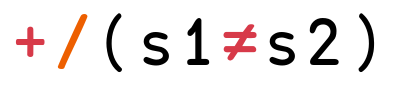We pass both arguments to the binary function `≠` (note that binary functions are always infix in APL). We then take the result of this operation and pass it to the unary function, in our case `+/`. Tracing the example of using the two strings `cats` and `city`, we get:⬆️ Run this Code

The B₁ combinator is a beautiful thing and is one of my favorite combinators. It was nicknamed the Blackbird by Raymond Smullyan. It implictly exists in the C++ solution. Whenever you use the `std::transform_reduce` algorithm, you provide two binary operations. The first will be combined with `std::reduce` to form a reduction. The reduction takes place after zipping the two sequences together using the second binary operation. If you squint hard, you can see the relationship between the APL and C++ soluiton.

It might is easier to see relationship between APL and C++ by looking at an in-between language, Haskell (for the Haskell uninitiated, `/=` is `not equal`)

``````import Data.Composition (.:) -- The B₁ Combinator

transformReduce reduceOp zipOp = foldl1 reduceOp .: zipWith zipOp
numDiffs = transformReduce (+) (/=)

numDiffs "cats" "city" -- 2
``````

Absolutely beautiful. Now, the Haskell expert will know that this doesn’t actually work. That is because `(/=)` returns a boolean and you can’t `sum` booleans. So the working Haskell solution is actually:

``````transformReduce reduceOp zipOp = foldl1 reduceOp .: zipWith zipOp
numDiffs = transformReduce (+) (fromEnum .: (/=))

numDiffs "cats" "city" -- 2
``````

⬆️ Run this Code

Would you look at that! Another use of the B₁ combinator. We need to convert the boolen to an integer, aka apply `fromEnum` (which does that conversion) after applying the `(/=)` binary operation, aka - the B₁ Combinator! So amazing!

And just to satisfy the Haskell programmer that is thinking in their head “that function wouldn’t be called `transformReduce` in Haskell” and “those argument names are too long” (which I agree), here you are:

``````zipFold f g = foldl1 f .: zipWith g
numDiffs = zipFold (+) (fromEnum .: (/=))

numDiffs "cats" "city" -- 2
``````

⬆️ Run this Code

I haven’t mentioned this till now, but in APL, they don’t refer to combinators as combinators. If it is function juxtaposition they are called trains, otherwise it is an operator. The two argument 2-train in APL is called `atop`, but there is also an `atop` operator: `⍤`. So the following two are equivalent: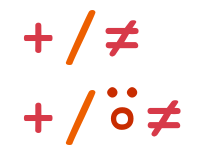This is also highlighted in my ARRAY 2022 paper Combinatory Logic and Combinators in Array Languages. The relevant excerpt from that paper highlights the B₁ Combinator in J and BQN as well, two other array languages.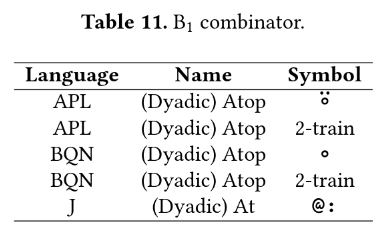## The ϕ₁ Combinator

### Problem 3: Uniquely Qualified

This problem is: given two lists, what elements only show up in one of the lists (not both). A basic solution in Python might look like the following:

``````def only_in_one_list(a, b):
res = []
for x in a:
if x not in b:
res.append(x)
for x in b:
if x not in a:
res.append(x)
return res
``````

⬆️ Run this Code

However, this solution once again violates my least favorite anti-pattern, ITM. A better and much more idiomatic Python solution is the following:

``````def only_in_one_list(a, b):
both = set(a) & set(b)
return [x for x in a + b if x not in both]
``````

⬆️ Run this Code

However, the solution in APL is the following: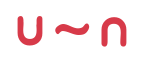This is an example of a “3-train” in APL. The `∪` reads `union`, the `~` reads `without`, and the `∩` reads intersection. So together, `∪~∩` reads `union without intersection`, which once again is a pretty good (if not amazing) description of what we are doing. And once again, the tricky (and beautiful) part of this solution is what a “3-train” is in APL. In our case where we will be passing 2 arguments to this function (`a` and `b`), it is the ϕ₁ combinator. The ϕ₁ combinator has the following evaluation (assuming are two arguments are `a` and `b`):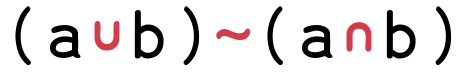We pass both arguments to the binary functions `∪` and `∩`. We then take the results of these operation and pass it to the middle binary function, in our case `~`. Tracing the example of using the two lists `1 2 3 4` and `3 4 5 6`, we get: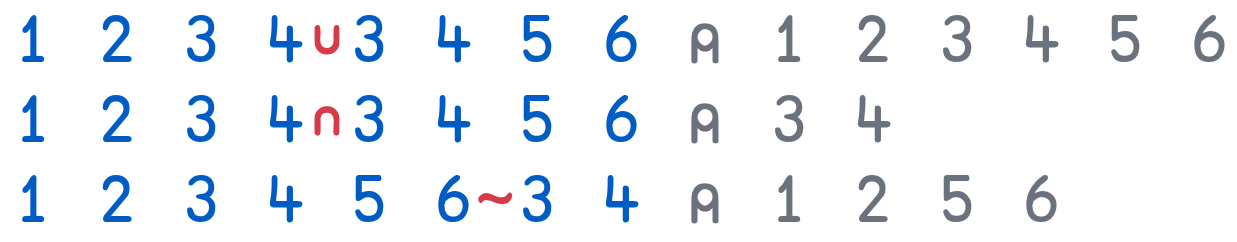⬆️ Run this Code

Although the ϕ combinator was given a nickname by Raymond Smullyan (the Phoenix), the ϕ₁ combinator wasn’t given a nickname … until recently that is. It was nicked the Pheasant in my aforementioned paper on combinators in array langauges.

In APL, 3-trains are called “forks.” Spefically, this is a dyadic fork because we are passing it two arguments. The Table 16 is the relevant table from my paper but it isn’t super interesting as every array language (APL, BQN & J) call it the same thing.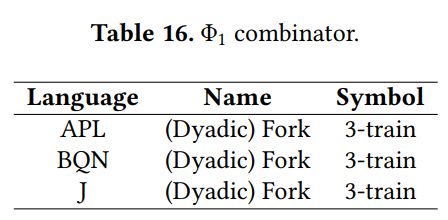## What is the point?

As Michael mentions in the ArrayCast podcast:

“It is like a line from a haiku…
there is something poetic about it…
it is very beautiful.”

This was in reference to the APL 3-train solution to Problem 3. However, it isn’t all about aesthetics.

I assert that combinators provide a greater opportunity for linear collection oriented programming, which is my favorite programming paradigm. Furthermore, I assert that in an collection oriented programming libraries such as:

Standard Libraries

Third Party Libraries

combinators can increase the opportunity for stream fusion and potentially index fusion as well. How far you could get with a library versus how far you could get with a compiler and new programming language is a question I hope to answer in the future.